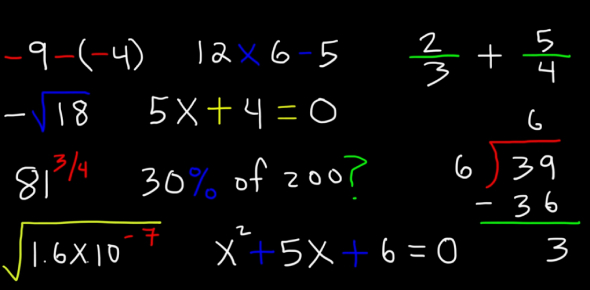# Just A Basic Maths Test Quiz!

10 Questions | Attempts: 1163
ShareSettingsMath is one of the subjects that most people fear when they are in school. Most people do not even practice enough to ensure that they understand the things they are taught. The quiz below is designed to test out your basic math skills. Do give it a shot and get to perfect them with more like it. All the best!

• 1.
What is the square root of 64?
• A.

8

• B.

6

• C.

12

• 2.
What is the square root of 36?
• A.

3

• B.

6

• C.

12

• 3.
What is the square root of 100?
• A.

25

• B.

5

• C.

10

• 4.
What is the square root of 25?
• A.

4

• B.

100

• C.

5

• 5.
What number comes next in the pattern? 2, 4, 8, 16, 32, 64....
• A.

128

• B.

96

• C.

120

• 6.
What number comes next in the pattern? 1, 3, 5, 7, 9, 11....
• A.

15

• B.

13

• C.

12

• 7.
What is 5 times 25?
• A.

120

• B.

100

• C.

125

• 8.
What is 1000 divided by 10?
• A.

10

• B.

100

• C.

1000

• 9.
What's the Pythagorean theorem?
• A.

A squared plus B squared= C squared

• B.

B squared minus A squared= C squared

• C.

C squared plus B squared= A squared

• 10.
What is the mode of these following numbers? 1, 4, 6, 2, 1, 1, 2, 7, 8, 9, 3, 3, 3, 3, 5, 5, 2, 3,
• A.

3

• B.

7

• C.

8

## Related TopicsBack to top
×

Wait!
Here's an interesting quiz for you.